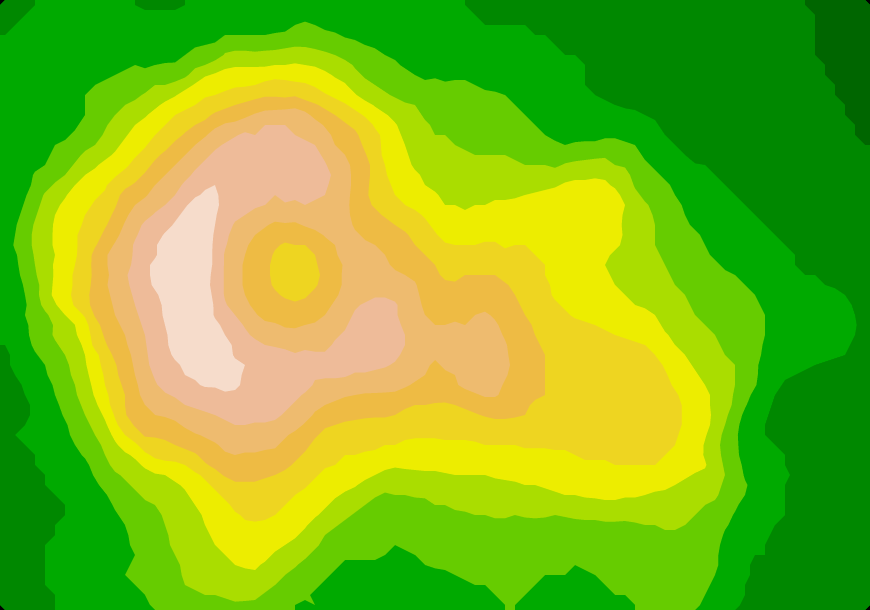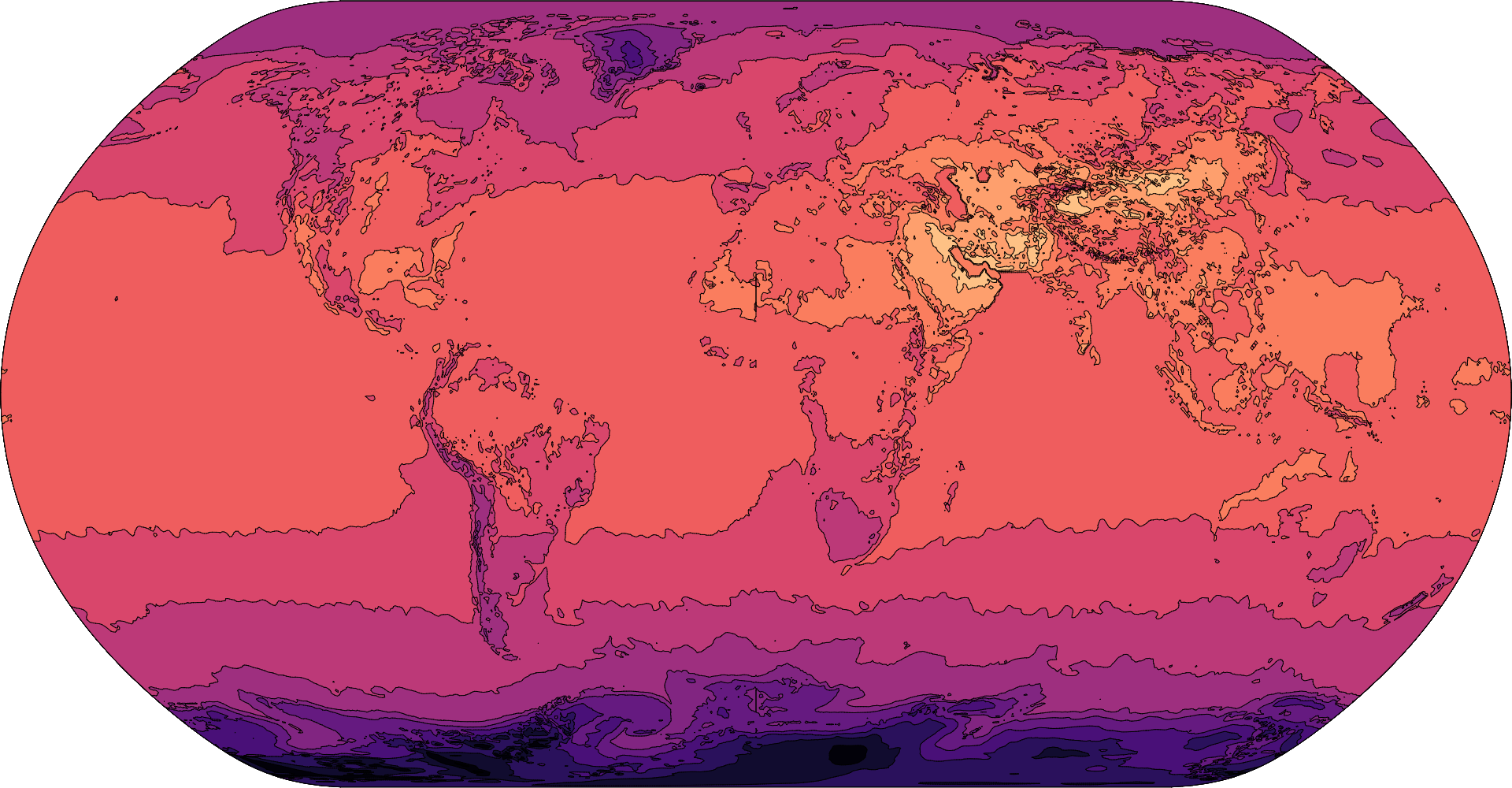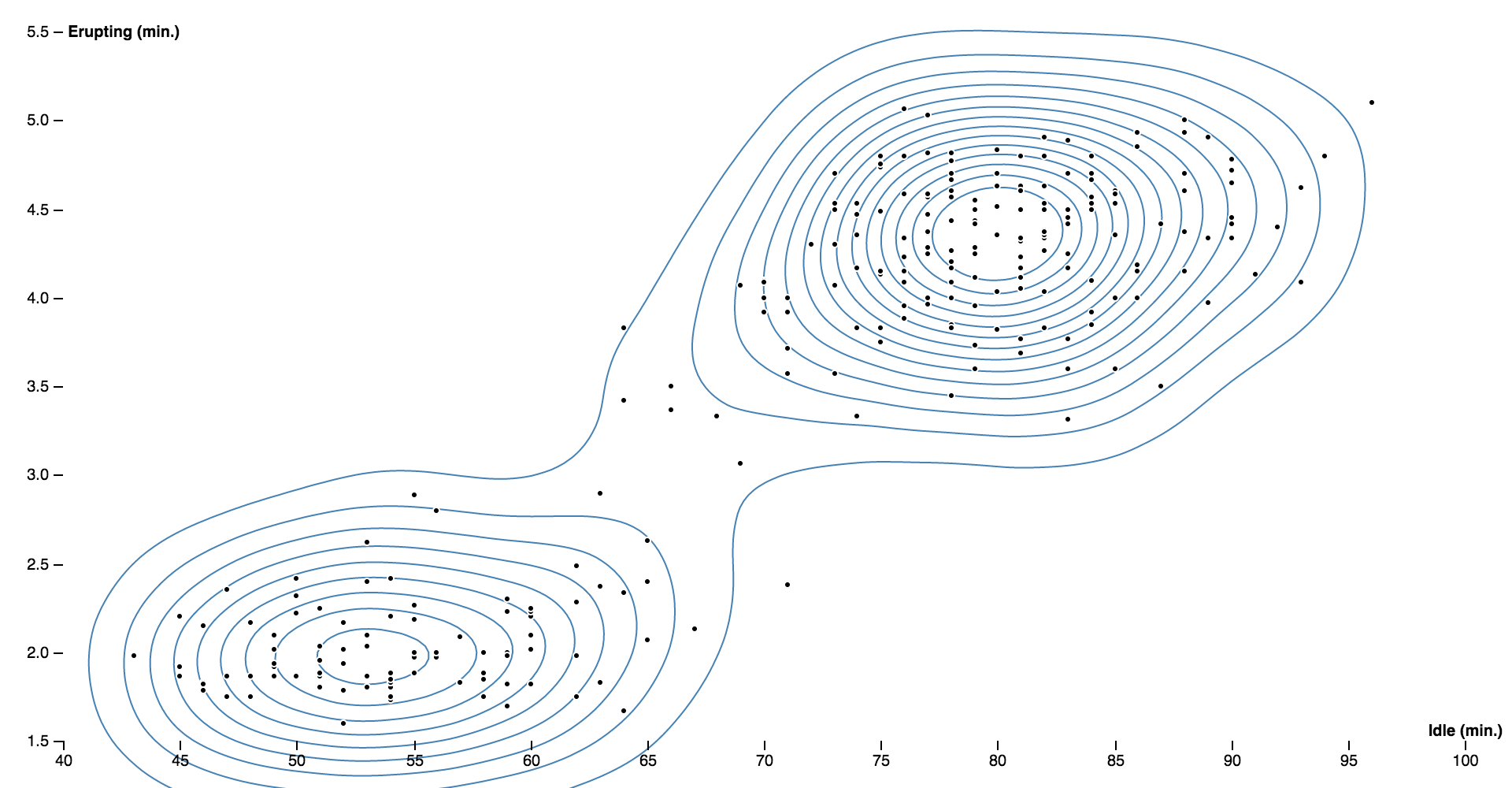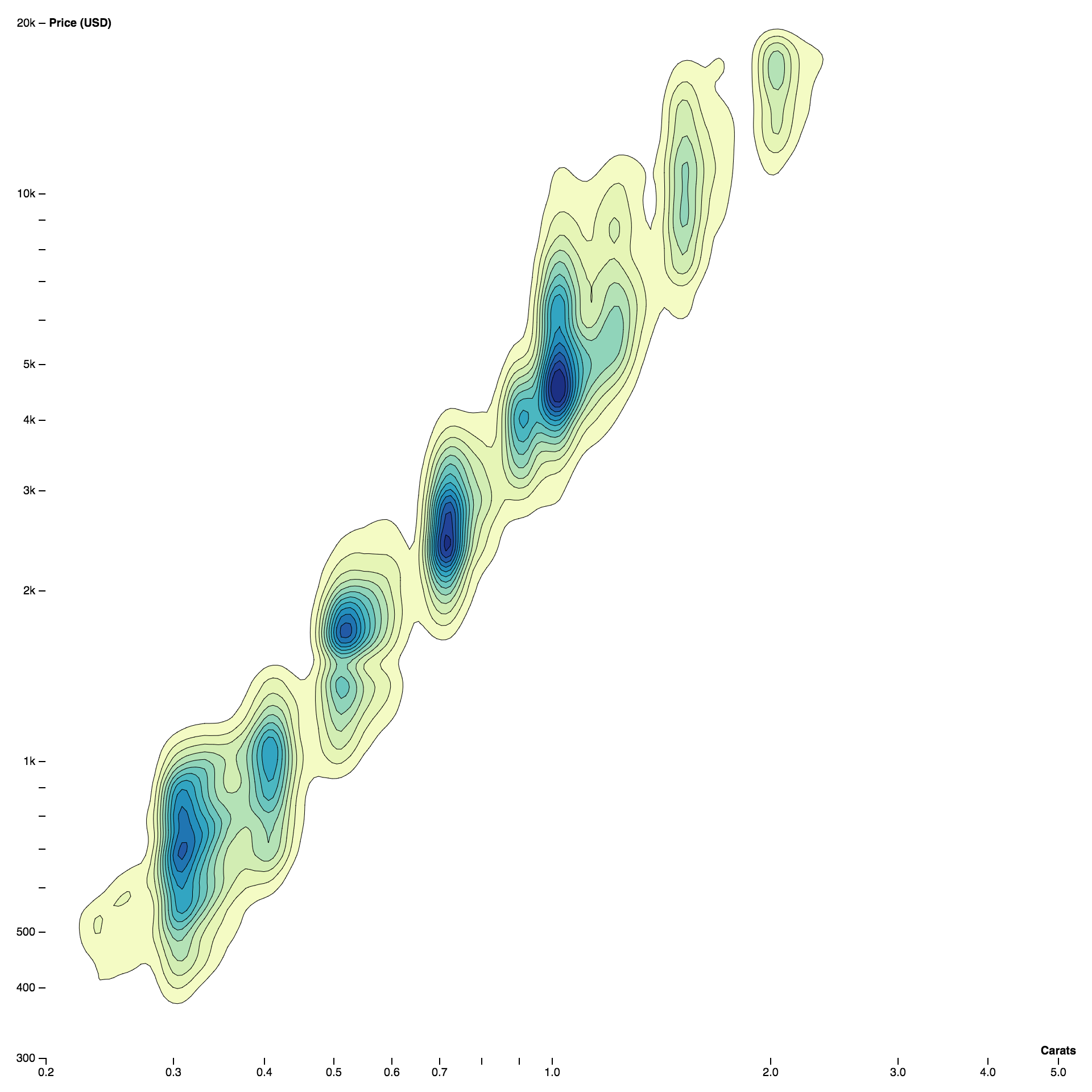# # d3-contour(opens new window)(opens new window)(opens new window)(opens new window)

### # Installing

`NPM` 安装: `npm install d3-contour`. 此外还可以下载 最新发行版 (opens new window). 可以直接从 d3js.org (opens new window)标准独立库 (opens new window) 或作为 D3 (opens new window) 的一部分直接引入. 支持 `AMD`, `CommonJS` 以及基础的标签引入形式. 如果使用标签引入, 则会暴露全局 `d3`:

``````<script src="https://d3js.org/d3-contour.v1.min.js"></script>
<script>

// Populate a grid of n×m values where -2 ≤ x ≤ 2 and -2 ≤ y ≤ 1.
var n = 256, m = 256, values = new Array(n * m);
for (var j = 0.5, k = 0; j < m; ++j) {
for (var i = 0.5; i < n; ++i, ++k) {
values[k] = goldsteinPrice(i / n * 4 - 2, 1 - j / m * 3);
}
}

// Compute the contour polygons at log-spaced intervals; returns an array of MultiPolygon.
var contours = d3.contours()
.size([n, m])
.thresholds(d3.range(2, 21).map(p => Math.pow(2, p)))
(values);

// See https://en.wikipedia.org/wiki/Test_functions_for_optimization
function goldsteinPrice(x, y) {
return (1 + Math.pow(x + y + 1, 2) * (19 - 14 * x + 3 * x * x - 14 * y + 6 * x * x + 3 * y * y))
* (30 + Math.pow(2 * x - 3 * y, 2) * (18 - 32 * x + 12 * x * x + 48 * y - 36 * x * y + 27 * y * y));
}

</script>
``````

### # API Reference

# d3.contours() <> (opens new window)

# contours(values) <> (opens new window)

``````var n = 256, m = 256, values = new Array(n * m);
for (var j = 0.5, k = 0; j < m; ++j) {
for (var i = 0.5; i < n; ++i, ++k) {
values[k] = goldsteinPrice(i / n * 4 - 2, 1 - j / m * 3);
}
}

function goldsteinPrice(x, y) {
return (1 + Math.pow(x + y + 1, 2) * (19 - 14 * x + 3 * x * x - 14 * y + 6 * x * x + 3 * y * y))
* (30 + Math.pow(2 * x - 3 * y, 2) * (18 - 32 * x + 12 * x * x + 48 * y - 36 * x * y + 27 * y * y));
}
``````

# contours.contour(values, threshold) <> (opens new window)

# contours.size([size]) <> (opens new window)

# contours.smooth([smooth]) <> (opens new window)

# contours.thresholds([thresholds]) <> (opens new window)

### # Density Estimation

# d3.contourDensity() <> (opens new window)

# density(data) <> (opens new window)

# density.x([x]) <> (opens new window)

``````function x(d) {
return d;
}
``````

# density.y([y]) <> (opens new window)

``````function y(d) {
return d;
}
``````

# density.weight([weight]) <> (opens new window)

``````function weight() {
return 1;
}
``````

# density.size([size]) <> (opens new window)

# density.cellSize([cellSize]) <> (opens new window)

# density.thresholds([thresholds]) <> (opens new window)

# density.bandwidth([bandwidth]) <> (opens new window)